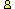CTK Exchange CTK Wiki Math Front Page Movie shortcuts Personal info Awards Terms of use Privacy Policy Cut The Knot! MSET99 Talk Games & Puzzles Arithmetic/Algebra Geometry Probability Eye Opener Analog Gadgets Inventor's Paradox Did you know?... Proofs Math as Language Things Impossible My Logo Math Poll Other Math sit's Guest book News sit's Recommend this siteCTK Exchange

 Subject: "Area Ratio With Vertex Next Side Midpoint Segments" Previous Topic | Next Topic
 ConferencesThe CTK ExchangeCollege mathTopic #709Printer-friendly copyEmail this topic to a friend Reading Topic #709
Bui Quang Tuan
Member since Jun-23-07
Mar-10-09, 11:03 AM (EST)"Area Ratio With Vertex Next Side Midpoint Segments"

 Dear All My Friends,Given convex quadrilateral ABCDE, F, G, H are midpoints of AB, BC, CD, DEM, N, P, Q are midpoints of AF, BG, CH, DEProve that Area(ABCD)/Area(MNPQ) = 8Best regards,Bui Quang Tuan

Alert | IP Printer-friendly page | Reply | Reply With Quote | Top
Bui Quang Tuan
Member since Jun-23-07
Mar-13-09, 09:06 AM (EST)1. "RE: Area Ratio With Vertex Next Side Midpoint Segments"
In response to message #0

 The Proof:Suppose R, S, T, U are midpoints of MN, NP, PQ, QM and X is midpoint of AC.Denote X //= Y as "X is parallel and equal Y".Because E, M, X are midpoints of AB, AF, AC so EM //= 1/2 BF and MX //= 1/2 FC, therefore E, M, X are collinear and M is midpoint of EX (1)Similarly G, P, X are collinear and P is midpoint of GX (2)From (1), (2) MP //= 1/2 EG.Moreover RS //= 1/2 MP then RS //= 1/4 EG (3)Similarly RU //= 1/4 FH (4)Note that RSTU and EFGH are Varignon's parallelograms of MNPQ and ABCDAlso note that area of the parallelogram can be calculated by two sides and angle between them or by two diagonals and angle between them.Based on (3), (4), by comparing of areas of two parallelograms RSTU and EFGH, we can show that:Area(RSTU) = 1/8 Area(EFGH)From this, of course Area(ABCD)/Area(MNPQ) = 8GENERALIZATIONIt is interesting that this fact can be some how generalized for any convex n gon A1A2A3... An. For it we define (n,k) gon of convex n gon. (n>=4, k>=1, k<=n-1)By one direction (for example clockwise) we connect each vertex of n gon A1A2... An with midpoint of side of next k sides from the vertex. We get n segments. By connecting all midpoints of these n segments we get one n gon and name it as (n, k) gon of given n gon A1A2A3... An.Of course by other direction (anti clockwise) we can get another (n, k) gon of n gon A1A2A3... An. So with given n gon and given k we can construct two (n, k) gons by two directions.With n>=5, I observer that ratio Area(n gon)/Area((n, k) gon) is not constant but:"two (n, k) gons of given convex n gon with given k obtain the same one area value"I still not have any idea to prove it.Best regards,Bui Quang Tuan

Alert | IP Printer-friendly page | Reply | Reply With Quote | TopalexbCharter Member
2342 posts
Mar-13-09, 09:18 AM (EST)2. "RE: Area Ratio With Vertex Next Side Midpoint Segments"
In response to message #1

 I expect the 4-gon problem to have a straightforward solution by linear algebra.If A, B, ... are looked at as vectors, then (1) the midpoints are (A + B)/2, ...(2) further midpoints are (2A + B + C)/4, ...If (U V W) is the area of triangle UVW then((U1 + U2) V W) = (U1 V W) + (U2 V W),(aU V W) = a(U V W),(U V W) = (V W U) = -(V U W),(U U W) = 0Splitting a quadrilateral into two triangles by a diagonal and using (2) and the properties of the area you should get the required result.Do not know yet about (n, k)-gon.Have this problem:There 2009 committees, each with 45 members, any two of which have exactly one person in common. Show that there is one person who is a member of all committees.

Alert | IP Printer-friendly page | Reply | Reply With Quote | Top

 Conferences | Forums | Topics | Previous Topic | Next Topic
 Select another forum or conference Lobby The CTK Exchange (Conference)   |--Early math (Public)   |--Middle school (Public)   |--High school (Public)   |--College math (Public)   |--This and that (Public)   |--Guest book (Protected)   |--Thoughts and Suggestions (Public) Educational Press (Conference)   |--No Child Left Behind (Public)   |--Math Wars (Public)   |--Mathematics and general education (Public)You may be curious to have a look at the old CTK Exchange archive.
Please do not post there.